# AP Calculus AB Practice Test 11

### Test Information10 questions20 minutes

Calculator Disallowed

1.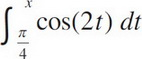=

2. What are the coordinates of the point of inflection on the graph of y = x3 - 15x2 + 33x + 100 ?

3. If 3x2 - 2xy + 3y = 1, then when x = 2,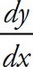=

4.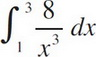=

5.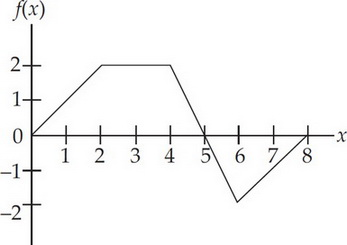The graph of a piecewise linear function f, for 0 ≤ x ≤ 8, is shown above. What is the value of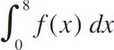?

6.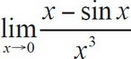7. If f(x) = x2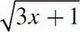, then f′(x) =

8. What is the instantaneous rate of change at t = -1 of the function f, if f(t) =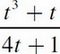?

9.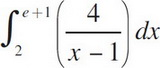=

10.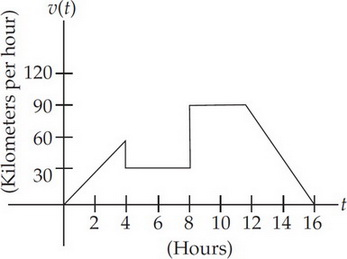A car's velocity is shown on the graph above. Which of the following gives the total distance traveled from t = 0 to t = 16 (in kilometers)?